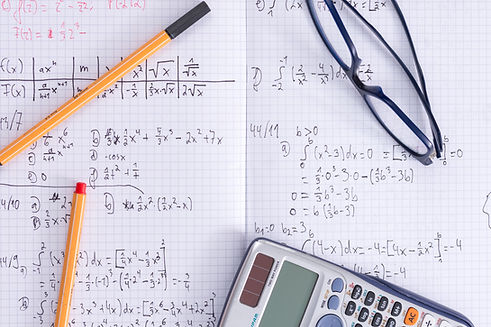top of page

## Math

Multiplication and Division

*Interpret a multiplication equation as a comparison,

*Multiply or divide to solve word problems involving multiplicative comparison, by using drawings and equations with a symbol for the unknown number to represent the problem,

*Solve multistep word problems posed with whole numbers and having whole-number answers.

*Find all factor pairs for a whole number in the range 1-100. Recognize that a whole number is a multiple of each of its factors.

*Generate a number or shape pattern that follows a given rule.

Place Value

*Recognize multi-digit whole number by applying concepts of place value and division.

*Read and write multi-digit whole numbers.

*Compare two multi-digit numbers based on meanings of the digits in each place.

*Use place value understanding to round multi-digit whole numbers.

* Fluently add and subtract multi-digit whole numbers using the standard algorithm.

*Multiply a whole number of up to four digits by a one-digit whole number, and multiply two two-digit numbers, using strategies based on place value and the properties of operations.

*Find whole-number quotients and remainders with up to four-digit dividends and one-digit divisors, using strategies based on place value.

Fractions

*Explain why a fraction is equivalent to a fraction.

*Compare two fractions with different numerators and different denominators.

*Understand addition and subtraction of fractions. -Understand addition and subtraction of fractions.

-Add and subtract mixed numbers with like denominators

-Solve word problems involving addition and subtraction of fractions.

-Apply understandings of multiplication to multiply of a fraction.

- Solve word problems involving multiplication of a fraction by a whole number.

-Express a fraction with denominator.

Decimals

- Use decimal notation for fractions with denominators.

- Compare two decimals to hundredths.

Measurement

- Know relative sizes of measurement units.

- Use the four operations to solve word problems using measurement.

-Represent measurement quantities using diagrams.

- Apply the area and perimeter formulas for  real world and mathematical problems.

- Make a line plot to display a data set of measurements. -Solve problems involving addition and subtraction of fractions by using information presented in line plots.

Geometry

- Recognize angles as geometric shapes.

-Measure angles in whole-number degrees using a protractor.

- Draw points, lines, line segments, rays, angles  and perpendicular and parallel lines.

-Identify line-symmetric figures and draw lines of symmetry.*Increased text complexity and growth of comprehension.

*Refer to details and examples when inferring information from text.

*Determine the meaning of words and phrases.

*Explain different meanings of words when reading different genres.

*Compare and contrast different views.

*By the end of the year read literature at the 4/5 grade level.

*Use phonics and decoding skills.

## Writing

*Write opinion pieces supporting a point of view.

Provides reasons that are supported by fact.

Provide a concluding statement.

*Write informative text to examine a topic.

*Write narratives to develop a real or imagined experience.

Produces writing that is clear and coherent and appropriate to the audience.

Uses technology with a significant command of keyboarding skills.

*Research Project## Speaking and Listening

*Engage in collaborative discussions.

*Paraphrases information.

*Identify key information being presented.

*Report on a topic- speaking clearly.

*Add audio recordings and visuals displays.## Language: Conventions, Effective Use and Vocabulary

*Demonstrate command of convention

use of grammar.

*Produces complete sentences.

*Use knowledge of language when writing speaking or listening.

*Uses phrases to convey ideas precisely.

*Use context as a clue for meaning.

Consult reference materials to find pronunciation.

*Demonstrate understanding of figurative language.bottom of page